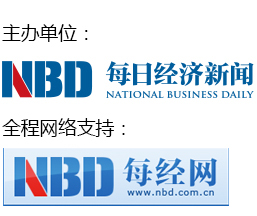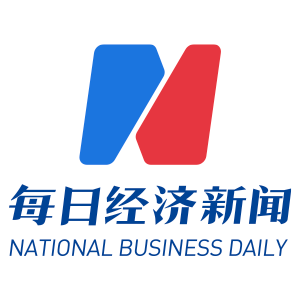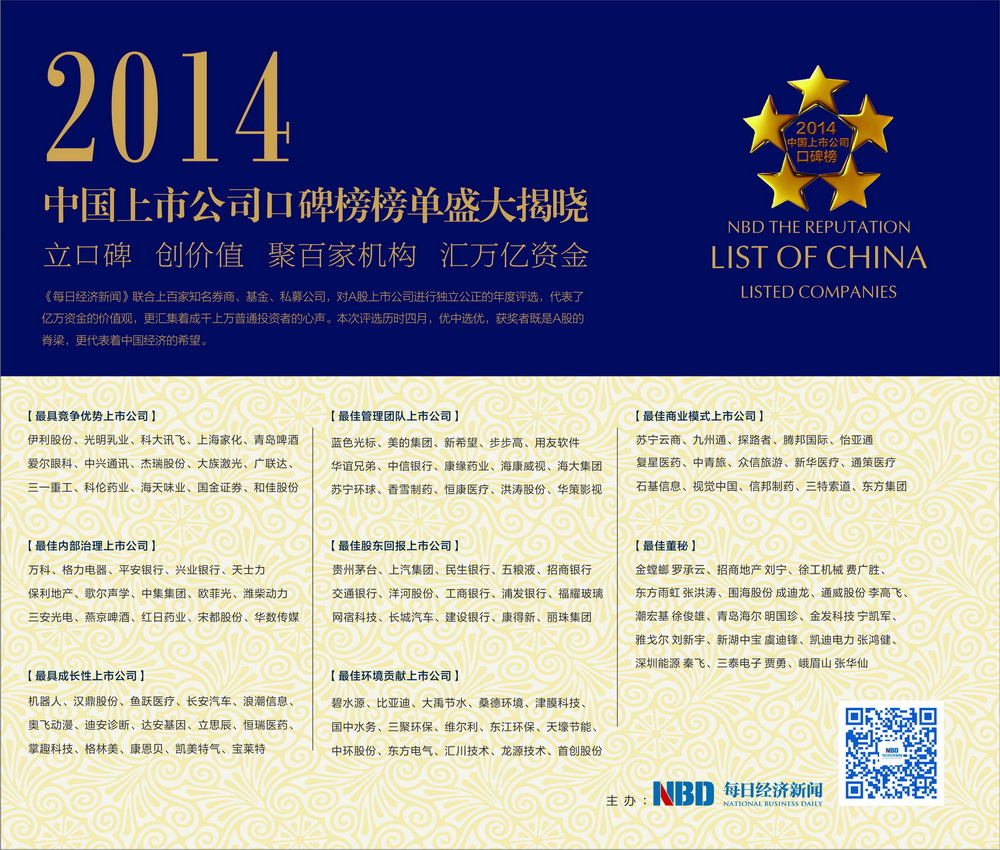“2014中国上市公司口碑榜”以八大维度全面考量A股市场，寻找中国最优秀上市公司。在三个多月的时间里，评选得到了线上线下万千投资者的参与和关注，代表数万亿资金的百家机构积极投票。今天，“2014中国上市公司口碑榜”评选结果正式出炉，共有120家上市公司荣获八项大奖，他们是中国经济最具活力和创新的希望之星，也是两千多家A股上市公司的精华所在。 【详情】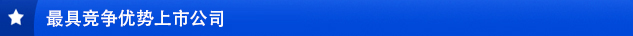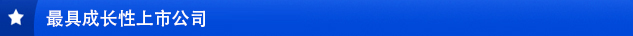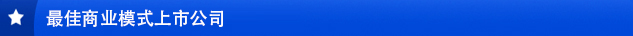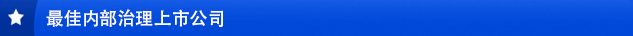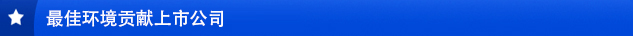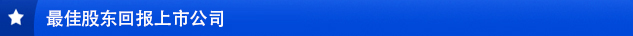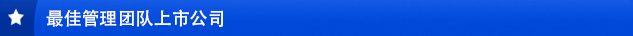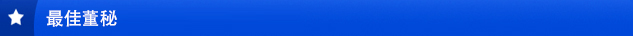## 开榜语

2014年，全球经济在缓慢复苏中暗流涌动，中国经济也步入了增速换挡期，经历着结构调整阵痛期。我们面临的，是空前复杂的经济形势。

2014年，A股市场也进入了一个最为纠结的时期。IPO大门再次打开，退市制度改革尘埃落定，沪港通横空出世，国资改革风生水起，这一切的一切，都在市场上掀起了一阵又一阵的涟漪，一次又一次的拍打着征战在资本市场第一线的“勇士”们。

2014年，近3000家上市公司各领风骚，但是真正撑起市场脊梁，能称之为优秀上市公司的，仅是其中的一小部分而已。因为一家公司是否优秀，不仅取决于发展的快慢或者盈利的多少，更在于包括广大投资者在内的各个市场主体，是否分享公司发展的喜悦与成果；因为一家公司是否优秀，不仅取决于获得各种奖项的多少，更在于是否获得包括广大投资者在内的各个市场主体，对公司发自内心的赞赏。

• 三一重工
• 伊利股份
• 国金证券
• 哈尔斯
• 上海家化
• 武汉健民
• 仁智油服
• 阳光电源
• 旋极信息
• 冠昊生物
• 獐子岛
• 聚飞光电
• 钢研高纳
• 四维图新
• 中兴通讯
• 吉鑫科技
• 圣农发展
• 全通教育
• 东阿阿胶
• 科大讯飞
• 和佳股份
• 科伦药业
• 美亚光电
• 光明乳业
• 广联达
• 青岛啤酒
• 华夏幸福
• 奥瑞金
• 秦川发展
• 爱尔眼科
• 普利特
• 大族激光
• 沃森生物
• 豪迈科技
• 棒杰股份
• 瑞丰光电
• 海天味业
• 松芝股份
• 中海科技
• 深天马A
• 宇通客车
• 国瓷材料
• 天广消防
• 杰瑞股份
• 南通锻压
• 莎普爱思
• 立讯精密
• 海信电器
• 华兰生物
• 索菲亚

• 中原环保
• 三聚环保
• 新纶科技
• 中国宝安
• 聚光科技
• 万向钱潮
• 兴蓉投资
• 龙源技术
• 津膜科技
• 华西能源
• 天顺风能
• 科达洁能
• 开能环保
• 川投能源
• 东港股份
• 宝新能源
• 置信电气
• 开山股份
• 中电环保
• 奥 特 迅
• 达实智能
• 先河环保
• 东江环保
• 雪迪龙
• 智慧能源
• 大禹节水
• 天壕节能
• 东方电气
• 赛为智能
• 国中水务
• 比亚迪
• 合康变频
• 桑德环境
• 维尔利
• 雪浪环境
• 国祯环保
• 碧水源
• 中环股份
• 天奇股份
• 双良节能
• 创业环保
• 首创股份
• 威孚高科
• 欣旺达
• 双塔食品
• 金通灵
• 汇川技术
• 巴安水务
• 南方汇通
• 多氟多

• 加加食品
• 尔康制药
• 三星电气
• 平安银行
• 三安光电
• 海南航空
• 水晶光电
• 歌尔声学
• 万科
• 亿利能源
• 燕京啤酒
• 洽洽食品
• 力帆股份
• 潍柴动力
• 格力电器
• 回天新材
• 翰宇药业
• 上海佳豪
• 欧菲光
• 中集集团
• 保利地产
• 西部证券
• 朗姿股份
• 华数传媒
• 广汽集团
• 宋城演艺
• 马应龙
• 顺鑫农业
• 宋都股份
• 贵州百灵
• 方正证券
• 中央商场
• 宏图高科
• 天士力
• 新华保险
• 兴业银行
• 红日药业
• 天地源
• 深振业
• 片仔癀
• 华谊嘉信
• 七匹狼
• 陕西煤业
• 汉得信息
• 新海宜
• 劲嘉股份
• 远光软件
• 益生股份
• 太阳纸业
• 鸿达兴业

• 瑞茂通
• 广誉远
• 探路者
• 中青旅
• 骅威股份
• 众信旅游
• 创意信息
• 新华医疗
• 博腾股份
• 三特索道
• 石基信息
• 信邦制药
• 苏宁云商
• 海特高新
• 腾邦国际
• 北京利尔
• 九州通
• 伟星新材
• 怡亚通
• 林洋电子
• 美盈森
• 友阿股份
• 海虹控股
• 上海钢联
• 桑乐金
• 银江股份
• 四川长虹
• 复星医药
• 九阳股份
• 韶能股份
• 通策医疗
• 生意宝
• 同方国芯
• 开元投资
• 南都电源
• 奥马电器
• 世联行
• 朗玛信息
• 方直科技
• 互动娱乐
• 金证股份
• 延华智能
• 盛和资源
• 长青股份
• 川大智胜
• 珈伟股份
• 视觉中国
• 高鸿股份
• 九安医疗
• 东方集团

• 浪潮软件
• 恒康医疗
• 莱宝高科
• 华侨城
• 蓝色光标
• 新华都
• 百视通
• 美的集团
• 东湖高新
• 步步高
• 香雪制药
• 海康威视
• 宗申动力
• 用友软件
• 仟源制药
• 荣盛发展
• 大湖股份
• 万润科技
• 海大集团
• 新希望
• 四川路桥
• 北大医药
• 中信银行
• 北京银行
• 海马汽车
• 苏宁环球
• 苏大维格
• 金风科技
• 特锐德
• 均胜电子
• 东北制药
• 洲明科技
• 江海股份
• 华策影视
• 光一科技
• 华夏银行
• 惠博普
• 华谊兄弟
• 润和软件
• 合肥三洋
• 天齐锂业
• 升达林业
• 洪涛股份
• 喜临门
• 智飞生物
• 顺网科技
• 华邦颖泰
• 东方网力
• 煌上煌
• 康缘药业

• 长城汽车
• 天舟文化
• 皖通高速
• 上海凯宝
• 胜利精密
• 浦发银行
• 三诺生物
• 贵州茅台
• 天汽模
• 浙江富润
• 汇金股份
• 万昌科技
• 建设银行
• 三维丝
• 五粮液
• 红旗连锁
• 蒙草抗旱
• 洋河股份
• 上汽集团
• 康得新
• 美盛文化
• 大连重工
• 福耀玻璃
• 金新农
• 誉衡药业
• 招商银行
• 佛慈制药
• 荣之联
• 登海种业
• 民生银行
• 和而泰
• 利君股份
• 交通银行
• 众生药业
• 丽珠集团
• 汉森制药
• 恒宝股份
• 网宿科技
• 聚龙股份
• 晨鸣纸业
• 海亮股份
• 工商银行
• 日发精机
• 科华生物
• 龙泉股份
• 海思科
• 奇正藏药
• 鑫科材料
• 星宇股份
• 赢时胜

• 金丰投资
• 隆基股份
• 科力远
• 汉鼎股份
• 金龙机电
• 掌趣科技
• 利亚德
• 浪潮信息
• 泰格医药
• 迪威视讯
• 环旭电子
• 四川九洲
• 恒瑞医药
• 国睿科技
• 奥飞动漫
• 华东医药
• 立思辰
• 鱼跃医疗
• 康恩贝
• 宝莱特
• 格林美
• 成飞集成
• 太极股份
• 丹甫股份
• 新筑股份
• 长安汽车
• 东旭光电
• 乐视网
• 软控股份
• 理邦仪器
• 亚太股份
• 机器人
• 新开普
• 东方通
• 达安基因
• 莱美药业
• 迪安诊断
• 万丰奥威
• 法拉电子
• 爱康科技
• 邦讯技术
• 金运激光
• 金花股份
• 银轮股份
• 凯美特气
• 卫士通
• 物产中拓
• 东软载波
• 大立科技
• 众和股份

• 神州泰岳 黄松浪
• 凯迪电力 张鸿健
• 围海股份 成迪龙
• 长江证券 徐锦文
• 通威股份 李高飞
• 深圳能源 秦飞
• 大华股份 吴坚
• 我武生物 王新华
• 彩虹股份 龙涛
• 渤海轮渡 宁武
• 东方航空 汪健
• 恩华药业 段保州
• 鹏博士 任春晓
• 双鹭药业 梁淑洁
• 平高电气 许明圣
• 晶盛机电 陆晓雯
• 齐心文具 沈焰雷
• 潮宏基 徐俊雄
• 青岛海尔 明国珍
• 金螳螂 罗承云
• 福瑞股份 林欣
• 万马股份 方春英
• 东方雨虹 张洪涛
• 徐工机械 费广胜
• 浙大网新 许克菲
• 金发科技 宁凯军
• 迪马股份 张爱明
• 峨眉山 张华仙
• 泸州老窖 曾颖
• 三泰电子 贾勇
• 中弘股份 金洁
• 新湖中宝 虞迪锋
• 山西汾酒 李秋喜
• 华业地产 赵双燕
• 海宁皮城 孙宇民
• 招商地产 刘宁
• 海南海药 张晖
• 紫江企业 高军
• 华平股份 奚峰伟
• 永辉超市 张经仪
• 蒙发利 李巧巧
• 滨江集团 李渊
• 奋达科技 谢玉平
• 雅戈尔 刘新宇
• 广田股份 朱旭
• 博彦科技 韩超
• 富临运业 黎昌军
• 光大银行 蔡允革
• 西南证券 徐鸣镝
• 中天城投 谭忠游

## 评选规则及流程

1、8月13日启动“2014中国上市公司口碑榜”活动，开启公众提名环节。

2、公众投资者通过每经官网或淘股吧进行提名，提名公司需标明具体奖项和理由，提名不限ID。

3、机构评委通过记者完成提名。

4、提名截止时间8月31日，综合机构提名与公众提名，汇总产生候选名单，每个榜单入围50家上市公司，在报纸上公布。

1、8月31日，候选名单出炉，进入投票环节。

2、公众投资者通过每经官网（www.nbd.com.cn）网站投票，每个奖项选出最多15家上市公司，每人只能投一次。

3、机构评委通过匿名通道投票，同时享有重点推荐三家公司的权利。

4、除网络投票通道外，同时开通邮寄选票的投票方式，选票将刊登在《每日经济新闻》。

1、会务组汇总各方投票，统计出投票结果，确定获奖企业名单。

2、邀请机构评委及获奖企业参加上市公司口碑榜颁奖典礼。

3、11月底，上市公司口碑被落地活动举行。

《每日经济新闻报社》社会监督热线电话400 889 0008